$$\newcommand{\id}{\mathrm{id}}$$ $$\newcommand{\Span}{\mathrm{span}}$$ $$\newcommand{\kernel}{\mathrm{null}\,}$$ $$\newcommand{\range}{\mathrm{range}\,}$$ $$\newcommand{\RealPart}{\mathrm{Re}}$$ $$\newcommand{\ImaginaryPart}{\mathrm{Im}}$$ $$\newcommand{\Argument}{\mathrm{Arg}}$$ $$\newcommand{\norm}{\| #1 \|}$$ $$\newcommand{\inner}{\langle #1, #2 \rangle}$$ $$\newcommand{\Span}{\mathrm{span}}$$

# 2.3e: Exercises - Transformations

$$\newcommand{\vecs}{\overset { \rightharpoonup} {\mathbf{#1}} }$$ $$\newcommand{\vecd}{\overset{-\!-\!\rightharpoonup}{\vphantom{a}\smash {#1}}}$$$$\newcommand{\id}{\mathrm{id}}$$ $$\newcommand{\Span}{\mathrm{span}}$$ $$\newcommand{\kernel}{\mathrm{null}\,}$$ $$\newcommand{\range}{\mathrm{range}\,}$$ $$\newcommand{\RealPart}{\mathrm{Re}}$$ $$\newcommand{\ImaginaryPart}{\mathrm{Im}}$$ $$\newcommand{\Argument}{\mathrm{Arg}}$$ $$\newcommand{\norm}{\| #1 \|}$$ $$\newcommand{\inner}{\langle #1, #2 \rangle}$$ $$\newcommand{\Span}{\mathrm{span}}$$ $$\newcommand{\id}{\mathrm{id}}$$ $$\newcommand{\Span}{\mathrm{span}}$$ $$\newcommand{\kernel}{\mathrm{null}\,}$$ $$\newcommand{\range}{\mathrm{range}\,}$$ $$\newcommand{\RealPart}{\mathrm{Re}}$$ $$\newcommand{\ImaginaryPart}{\mathrm{Im}}$$ $$\newcommand{\Argument}{\mathrm{Arg}}$$ $$\newcommand{\norm}{\| #1 \|}$$ $$\newcommand{\inner}{\langle #1, #2 \rangle}$$ $$\newcommand{\Span}{\mathrm{span}}$$

### A: Concepts

Exercise $$\PageIndex{A}$$

1) When examining the formula of a function that is the result of multiple transformations, how can you tell a horizontal shift from a vertical shift?
2) When examining the formula of a function that is the result of multiple transformations, how can you tell a horizontal stretch from a vertical stretch?
3) When examining the formula of a function that is the result of multiple transformations, how can you tell a horizontal compression from a vertical compression?
4) When examining the formula of a function that is the result of multiple transformations, how can you tell a reflection with respect to the $$x$$-axis from a reflection with respect to the $$y$$-axis?
5) How can you determine whether a function is odd or even from the formula of the function?

1. A horizontal shift results when a constant is added to or subtracted from the input. A vertical shifts results when a constant is added to or subtracted from the output.

3. A horizontal compression results when a constant greater than $$1$$ is multiplied by the input. A vertical compression results when a constant between $$0$$ and $$1$$ is multiplied by the output.

5. For a function $$f$$, substitute (−x) for (x) in $$f(x)$$. Simplify. If the resulting function is the same as the original function, $$f(−x)=f(x)$$, then the function is even. If the resulting function is the opposite of the original function, $$f(−x)=−f(x)$$, then the original function is odd. If the function is not the same or the opposite, then the function is neither odd nor even.

### B: Describe transformations of a function written in function notation

Exercise $$\PageIndex{B}$$

$$\bigstar$$ Describe how the graph of the function is a transformation of the graph of the original function $$f$$.

 6) $$y=f(x−49)$$ 7) $$y=f(x+43)$$ 8) $$y=f(x+3)$$ 9) $$y=f(x-4)$$ 10) $$y=f(x)+5$$ 11) $$y=f(x)+8$$ 12) $$y=f(x)−2$$ 13) $$y=f(x)−7$$ 14) $$y=f(x−2)+3$$ 15) $$y=f(x+4)-1$$ 16) $$g(x)=f(−x)$$ 17) $$g(x)=−f(x)$$ 18) $$g(x)=6f(x)$$ 19) $$g(x)=4f(x)$$ 20) $$g(x)=f(2x)$$ 21) $$g(x)=f(5x)$$ 22) $$g(x)=f \left(\tfrac{1}{5}x \right)$$ 23) $$g(x)=f \left(\tfrac{1}{3}x \right)$$ 24) $$g(x)=−f(3x)$$ 25) $$g(x)=3f(−x)$$

7. The graph of $$f(x+43)$$ is a horizontal shift to the left $$43$$ units of the graph of $$f$$.

9. The graph of $$f(x-4)$$ is a horizontal shift to the right $$4$$ units of the graph of $$f$$.

11. The graph of $$f(x)+8$$ is a vertical shift up $$8$$ units of the graph of $$f$$.

13. The graph of $$f(x)−7$$ is a vertical shift down $$7$$ units of the graph of $$f$$.

15. The graph of $$f(x+4)−1$$ is a horizontal shift left $$4$$ units and vertical shift down $$1$$ unit of the graph of $$f$$.

17. The graph of $$g$$ is a vertical reflection (across the x-axis) of the graph of $$f$$.

19. The graph of $$g$$ is a vertical stretch by a factor of 4 of the graph of $$f$$.

21. The graph of $$g$$ is a horizontal compression by a factor of $$\frac{1}{5}$$ of the graph of $$f$$.

23. The graph of $$g$$ is a horizontal stretch by a factor of 3 of the graph of $$f$$.

25. The graph of $$g$$ is a horizontal reflection across the y-axis and a vertical stretch by a factor of 3 of the graph of $$f$$.

### C: Graph transformations of a basic function

Exercise $$\PageIndex{C}$$

$$\bigstar$$ Begin by graphing the basic quadratic function $$f(x)=x^2$$. State the transformations needed to apply to $$f$$ to graph the function below. Then use transformations to graph the function.

 27. $$g(x) = x^{2} + 1$$ 28. $$g(x) = x^{2} − 4$$ 29. $$g(x) = (x − 5)^{2}$$ 30. $$g(x) = (x + 1)^{2}$$ 31. $$g(x) = (x − 5)^{2} + 2$$ 32. $$g(x) = (x + 2)^{2} − 5$$ 33. $$f(t)=(t+1)^2−3$$ 34. $$f ( x ) = - ( x + 2 ) ^ { 2 }$$ 35. $$f ( x ) = - x ^ { 2 } + 6$$  36. $$g ( x ) = - 2 x ^ { 2 }$$ 37. $$g(x)=4(x+1)^2−5$$ 38. $$g(x)=5(x+3)^2−2$$ 39. $$h ( x ) = \tfrac { 1 } { 2 } ( x - 1 ) ^ { 2 }$$ 40. $$h ( x ) = \tfrac { 1 } { 3 } ( x + 2 ) ^ { 2 }$$ 41. $$f ( x ) = ( -\tfrac{1}{2}x - 3 ) ^ 2 + 1$$  42. $$g(x)=(-2x+3)^2 -4$$

$$\bigstar$$ Begin by graphing the square root function $$f(x)=\sqrt{x}$$. State the transformations needed to apply to $$f$$ to graph the function below. Then use transformations to graph the function.

 43. $$g(x) = \sqrt{x} − 5$$ 44. $$g(x) = \sqrt{x − 5}$$ 45. $$g(x) = \sqrt{x − 2} + 1$$ 46. $$g(x) = \sqrt{x + 2} + 3$$ 47. $$a(x)=\sqrt{−x+4}$$ 48. $$m(t)=3-\sqrt{t+2}$$ 49. $$h ( x ) = \sqrt { - x } + 2$$ 50. $$g ( x ) = - \sqrt { x } + 2$$ 51. $$g ( x ) = - \frac { 1 } { 2 } \sqrt { x - 3 }$$ 52. $$h ( x ) = - \sqrt { x - 2 } + 1$$ 53. $$f ( x ) = 4 \sqrt { x - 1 } + 2$$ 54. $$f ( x ) = - 5 \sqrt { x + 2 }$$ 55.  $$k(x) = \sqrt{2x+5} - 1$$ 56.1 $$a(x) = \sqrt{\tfrac{1}{3} x - 4}$$ 56.2 $$b(x) = \sqrt{3-x}+2$$

$$\bigstar$$ Begin by graphing the absolute value function $$f(x)=| x |$$. State the transformations needed to apply to $$f$$ to graph the function below. Then use transformations to graph the function.

 57. $$h(x) = |x + 4|$$ 58. $$h(x) = |x − 4|$$ 59. $$h(x) = |x − 1| − 3$$ 60. $$h(x) = |x + 2| − 5$$ 61. $$g ( x ) = - | x - 1 |$$ 62. $$h(x)=|x−1|+4$$ 63. $$f ( x ) = - 3 | x |$$ 64. $$f ( x ) = - | x | - 3$$ 65. $$h(x)=−2|x−4|+3$$ 66. $$n(x)=\dfrac{1}{3}|x−2|$$ 67. $$h ( x ) = | - 3 x + 4 | - 2$$ 68. $$g(x) = | \tfrac{1}{3}x-2| + 1$$

$$\bigstar$$ Begin by graphing the standard cubic function $$f(x) = x^3$$. State the transformations needed to apply to $$f$$ to graph the function below. Then use transformations to graph the function.

 69. $$h(x) = (x − 2)^{3}$$ 70. $$h(x) = x^{3} + 4$$ 71. $$h(x) = (x − 1)^{3} − 4$$ 72. $$h(x) = (x + 1)^{3} + 3$$ 73. $$g ( x ) = - ( x + 2 ) ^ { 3 }$$ 74. $$k(x)=(x−2)^3−1$$ 75. $$g ( x ) = - x ^ { 3 } + 4$$ 76. $$m(x)=\tfrac{1}{2}x^3$$ 77. $$g ( x ) = - \frac { 1 } { 4 } ( x + 3 ) ^ { 3 } - 1$$ 78. $$q(x)=\big(\tfrac{1}{4}x\big)^3+1$$ 79. $$p(x)=\big(\tfrac{1}{3}x\big)^3−3$$

$$\bigstar$$ Begin by graphing the appropriate parent function : the basic cube root function $$f(x)=\sqrt{x}$$,  constant function $$f(x)=0$$,  or linear function $$f(x)=x$$. Then use transformations of this graph to graph the given function.

 81. $$g( x ) = \sqrt [ 3 ] {x} -1$$ 82. $$g( x ) = \sqrt [ 3 ] { x - 1 }$$ 83. $$g( x ) = \sqrt [ 3 ] { x - 2 } + 6$$ 84. $$g( x ) = \sqrt [ 3 ] { x + 8 } - 4$$ 84.1 $$g( x ) = \sqrt [ 3 ] { -x + 3 } - 2$$ 84.1 $$g( x ) = - \sqrt [ 3 ] { x - 1 } + 2$$ 85. $$g ( x ) = -2 \sqrt [ 3 ] { x + 3 } + 4$$ 86. $$g ( x ) = \sqrt [ 3 ] {-2 x - 5 } - 1$$ 87. $$f(x) = x + 3$$ 88. $$h ( x ) = - 2 x + 1$$ 89. $$g(x) = −4$$

$$\bigstar$$ Begin by graphing the basic reciprocal function $$f(x)=\frac{1}{x}$$. State the transformations needed to apply to $$f$$ to graph the function below. Then use transformations to graph the function.

 91. $$f(x) = \dfrac{1}{x−2}$$ 92. $$f(x) = \dfrac{1}{x+3}$$ 93. $$f(x) = \dfrac{1}{x} + 5$$ 94. $$f(x) = \dfrac{1}{x} − 3$$ 95. $$f(x) = \dfrac{1}{x+1} − 2$$ 96. $$f(x) = \dfrac{1}{x−3} + 3$$ 97. $$f ( x ) = - \dfrac { 1 } { x + 2 }$$ 98. $$f ( x ) = - \dfrac { 1 } { x }$$ 99.$$p( x ) = - \dfrac { 1 } { x + 1 } + 2$$ 100.1 $$a(x) = \dfrac{2}{x-3} -5$$ 100.2 $$b(x) = \dfrac{1}{2x+6} +4$$
Answers to Odd Numbered Exercises for the Squaring Function:
Squaring Function
 27. $$y = x^{2}$$; Shift up $$1$$ unit; domain: $$ℝ$$; range: $$[1, ∞)$$$$g(x) = x^{2} + 1$$ 29. $$y = x^{2}$$; Shift right $$5$$ units; domain: $$ℝ$$; range: $$[0, ∞)$$$$g(x) = (x − 5)^{2}$$ 31. $$y = x^{2}$$; Shift right $$5$$ units and up $$2$$ units; domain: $$ℝ$$; range: $$[2, ∞)$$$$g(x) = (x − 5)^{2} + 2$$ 33.  Shift left $$1$$ unit and down $$3$$ units;$$f(t)=(t+1)^2−3$$ #35 Reflect over x-axis, up $$6$$ units.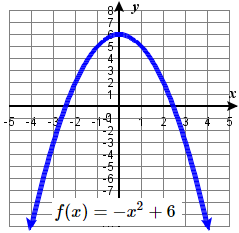37 $$f(x)=x^2$$ is shifted to the left $$1$$ unit, stretched vertically by a factor of $$4$$, and shifted down $$5$$ units.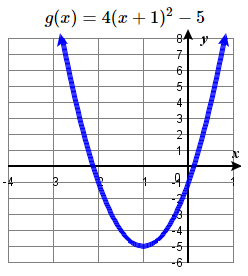39. Shift right $$1$$ unit, and vertically shrink by a factor of $$\frac{1}{2}$$$$h(x) = \frac { 1 } { 2 } ( x - 1 ) ^ { 2 }$$ #41 Shift right $$3$$, reflect over $$y$$-axis, horizontally stretch by a factor of $$2$$, up $$1$$ units.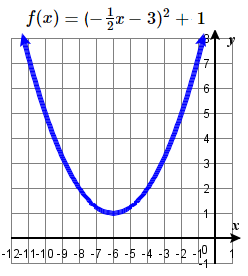for # 41, if $$f ( x ) = ( -\tfrac{1}{2}x - 3 ) ^ 2 + 1$$ is rewritten as  $$f ( x ) = ( -\tfrac{1}{2}(x + 6) ) ^ 2 + 1$$ , then the transformations would be horizontal stretch by a factor of 2, reflect in $$y$$-axis (no change), left 6, up 1.
Answers to Odd Numbered Exercises for the Square Root Function

Square Root Function

 43. $$y = \sqrt{x}$$; Shift down $$5$$ units; domain: $$[0, ∞)$$; range: $$[−5, ∞)$$$$g(x) = \sqrt{x} − 5$$ 45. $$y = \sqrt{x}$$; Shift right $$2$$ units and up $$1$$ unit; domain: $$[2, ∞)$$; range: $$[1, ∞)$$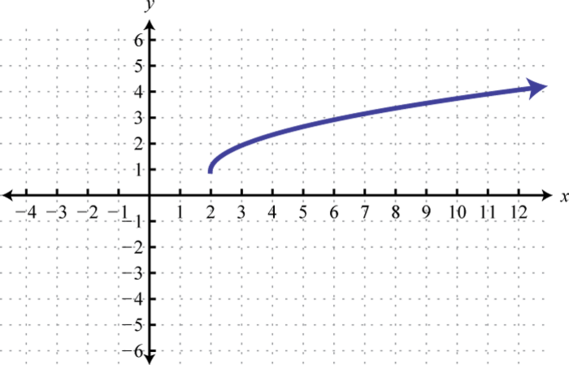$$g(x) = \sqrt{x − 2} + 1$$ 47 The graph of $$f(x)=\sqrt{x}$$ is shifted left $$4$$ units and then reflected across the $$y$$-axis.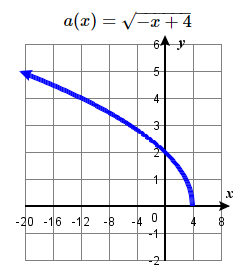49. Reflect over $$y$$-axis, up $$2$$$$h ( x ) = \sqrt { - x } + 2$$ 51. Right $$3$$, Reflect over $$x$$ axis, Vertically compressed by a factor of $$1/2$$.$$g ( x ) = - \frac { 1 } { 2 } \sqrt { x - 3 }$$ 53. Right 1, Vertically stretched by a factor of $$4$$, up $$2$$$$f(x) = 4 \sqrt { x - 1 } + 2$$ #55 Horizontal compression by $$1/2$$, shift left $$2.5$$, down $$1$$ unit.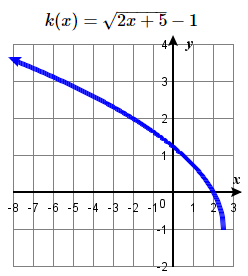Answers to Odd Numbered Exercises for the Absolute Value Function:

Absolute Value Function

 57. $$y = |x|$$; Shift left $$4$$ units; domain: $$ℝ$$; range: $$[0, ∞)$$$$h(x) = |x + 4|$$ . 59. $$y = |x|$$; Shift right $$1$$ unit and down $$3$$ units; domain: $$ℝ$$; range: $$[−3, ∞)$$$$h(x) = |x − 1| − 3$$ . 61. Right $$1$$, Reflect over $$x$$-axis$$g(x) = - | x - 1 |$$ .
 63. Reflect over $$x$$-axis, vertically stretch by a factor of $$3$$$$f(x) = - 3 | x |$$ 65 The graph of $$f(x)=|x|$$ is shifted horizontally $$4$$ units to the right, stretched vertically by a factor of $$2$$, reflected across the horizontal axis, then shifted up $$3$$ units.67. $$h(x) = |-3(x-\tfrac{4}{3})| -2$$ $$\longrightarrow$$ Horizontally compress by a factor of $$\tfrac{1}{3}$$, right $$\tfrac{4}{3}$$, down $$2$$Answers to Odd Numbered Exercises for the Cubing Function

Cubing Function

 69. $$y = x^{3}$$ ; Shift right $$2$$ units; domain: $$ℝ$$; range: $$ℝ$$$$h(x) = (x − 2)^{3}$$ 71. $$y = x^{3}$$; Shift right $$1$$ unit and down $$4$$ units; domain: $$ℝ$$; range: $$ℝ$$$$h(x) = (x − 1)^{3} − 4$$ 73. Left $$2$$ units, reflect over x-axis$$g(x) = - ( x + 2 ) ^ { 3 }$$
 75. Reflect over x-axis, up $$4$$ units$$g ( x ) = - x ^ { 3 } + 4$$ 77. Left $$3$$ units, reflect over x-axis, vertically shrink by a factor of $$\frac {1}{4}$$, down $$1$$ unit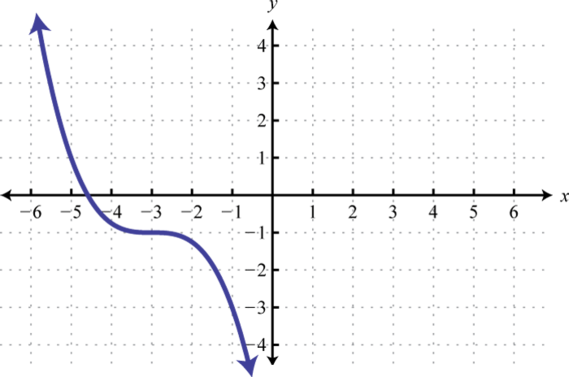$$g ( x ) = - \frac {1}{4} (x + 3) ^ {3} - 1$$ 79. Stretch horizontally by a factor of $$3$$ and shift vertically downward by $$3$$ units.Answers to Odd Numbered Exercises for the Cube Root, Linear and Constant Functions

Cube Root, Linear, Constant Functions

 81. $$y = \sqrt [ 3 ] { x }$$; Shift down $$1$$83. $$y = \sqrt [ 3 ] { x }$$; Shift up $$6$$ units and right $$2$$ units; domain: $$ℝ$$; range: $$ℝ$$85. $$y = \sqrt [ 3 ] { x }$$; Left $$3$$, reflect over $$x$$-axis, vertically stretch by a factor of $$2$$, up $$4$$.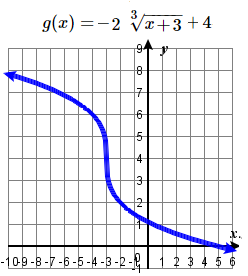87. $$y = x$$; Shift up $$3$$ units; domain: $$\mathbb{R}$$; range: $$\mathbb{R}$$$$f(x) = x + 3$$ 89. Basic graph $$y = −4$$; domain: $$ℝ$$; range: $$\{−4\}$$$$g(x) = −4$$
Answers to Odd Numbered Exercises for the Reciprocal Function:

Reciprocal Function

 91. $$y = \frac{1}{x}$$; Shift right $$2$$ units; domain: $$(−∞, 2) ∪ (2, ∞)$$; range: $$(−∞, 0) ∪ (0, ∞)$$$$f(x) = \frac{1}{x−2}$$ 93. $$y = \frac{1}{x}$$; Shift up $$5$$ units; domain: $$(−∞, 0) ∪ (0, ∞)$$; range: $$(−∞, 1) ∪ (1, ∞)$$$$f(x) = \frac{1}{x} + 5$$ $$\star$$ 95. $$y = \frac{1}{x}$$; Shift left $$1$$ unit and down $$2$$ units; domain: $$(−∞, −1) ∪ (−1, ∞)$$; range: $$(−∞, −2) ∪ (−2, ∞)$$$$f(x) = \frac{1}{x+1} − 2$$ 97
 97. Left $$2$$ units, reflect over x-axis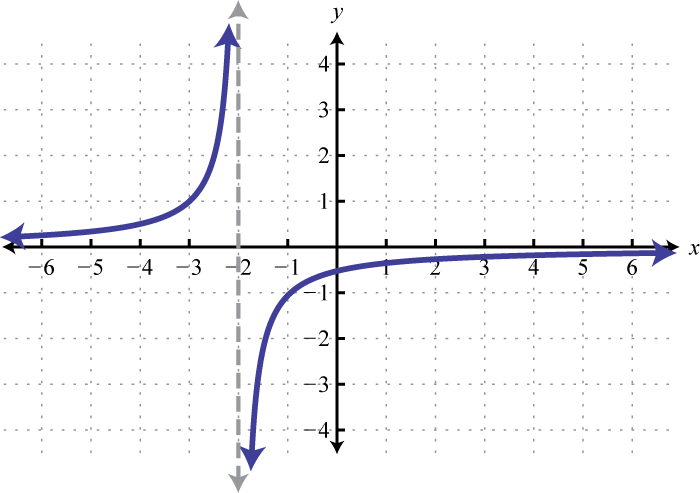$$f ( x ) = - \frac { 1 } { x + 2 }$$ #99  Left $$1$$ unit, reflect over x-axis, up $$2$$ units.### D: Graph Transformations of a Graph

Exercise $$\PageIndex{D}$$

$$\bigstar$$ Use the graph of $$f(x)$$ shown in the Figure below to sketch a graph of each transformation of $$f(x)$$.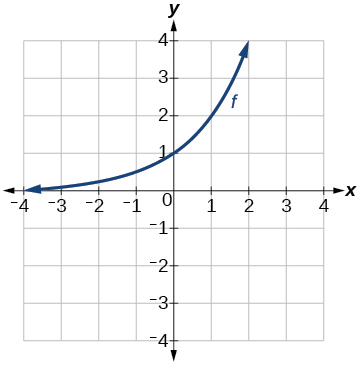Given the graph of  $$f(x)$$ on the right, sketch the graph for the following transformations of $$f$$   101. $$h(x)=2^x-3$$ 102. a) $$g(x)=2^x+1$$        b) $$w(x)=2^x−1$$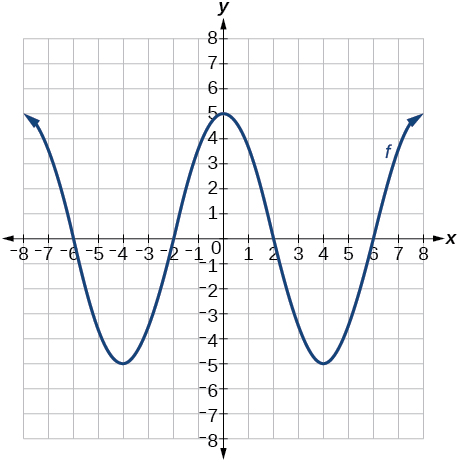Given the graph of  $$f(x)$$ on the right, sketch the graph for the following transformations of $$f$$   103. a) $$g(x)=−f(x)$$        b) $$g(x)=f(x−2)$$ 104. a) $$g(x)=f(x)−2$$        b) $$g(x)=f(x+1)$$

106. Given the graph of  $$f(x)$$ below, sketch the graph for the following transformations of $$f$$

 $$a(x) = f(x)+1$$ $$b(x) = f(x+1)$$ $$c(x) = f(x)+2$$ $$d(x) = -f(x)$$ $$e(x) = f(1-x)-2$$ $$f(x) = 2f(x)$$ $$g(x) = -f(x)$$ $$h(x) = \tfrac{1}{2} f(x+2)+3$$ $$i(x) = f(x)-1$$ $$j(x) = f(x-1)$$ $$k(x) = f(x)-2$$ $$l(x) = f(-x)$$ $$m(x) = -f(x-1)+2$$ $$n(x) = f(2x)$$ $$o(x) = f(-x)$$ $$p(x) = f( \tfrac{1}{2} x-2)-3$$101103. a103. b### E: Match transformations of functions with graphs

Exercise $$\PageIndex{E}$$

$$\bigstar$$ Match the graph to the function definition.

 107. $$f(x) = \sqrt{x + 4}$$ 108. $$f(x) = |x − 2| − 2$$ 109. $$f(x) = \sqrt{x + 1} -1$$ 110. $$f(x) = |x − 2| + 1$$ 111. $$f(x) = \sqrt{x + 4} + 1$$ 112. $$f(x) = |x + 2| − 2$$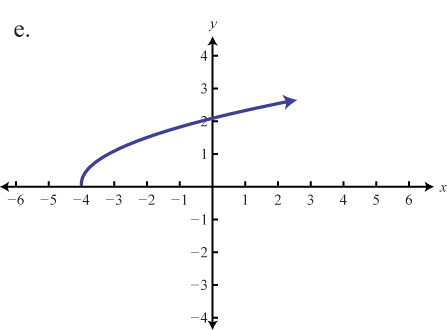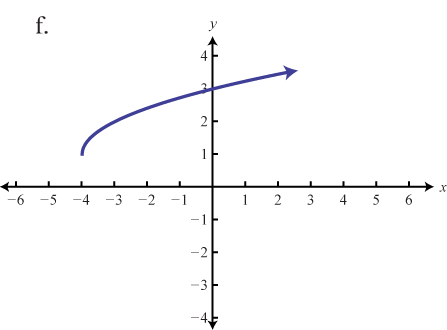Match the graph to the given function definition.

 113. $$f ( x ) = - 3 | x |$$ 114. $$f ( x ) = - ( x + 3 ) ^ { 2 } - 1$$ 115. $$f ( x ) = - | x + 1 | + 2$$ 116. $$f ( x ) = - x ^ { 2 } + 1$$ 117. $$f ( x ) = - \frac { 1 } { 3 } | x |$$ 118. $$f ( x ) = - ( x - 2 ) ^ { 2 } + 2$$Figure (a)Figure (b) $$\star$$Figure (c)Figure (d) $$\star$$Figure (e)Figure (f) $$\star$$

part 1 answers 107e, 109d, 111f,    part 2 Answers: 113.b, 115.d, 117.f

### F: Construct equations from graphs of transformed basic functions

Exercise $$\PageIndex{F}$$

$$\bigstar$$ Write an equation for each graphed function by using transformations of the graphs of one of the toolkit functions.

 119.120.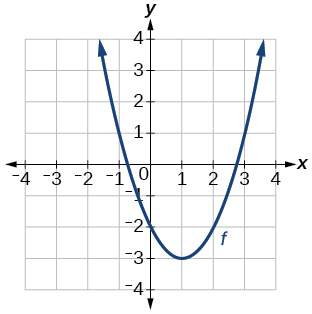121.122.123.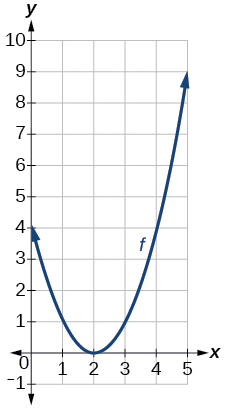124.125.126.127.128.129.130. (a)130. (b)130. (c)119. $$f(x)=|x-3|−2$$  121. $$f(x)=\sqrt{x+3}−1$$ 123. $$f(x)=(x-2)^2$$ 125.  $$f(x)=|x+3|−2$$ 127. $$f(x)=−\sqrt{x}$$ 129. $$f(x)=−(x+1)^2+2$$

$$\bigstar$$ Write an equation that represents the function whose graph is given.

 131.132.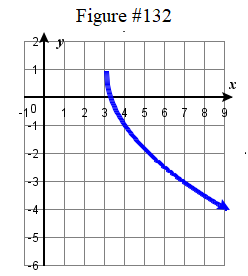133.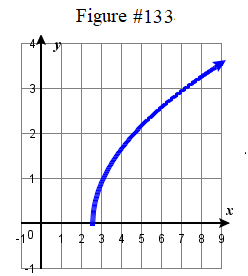134.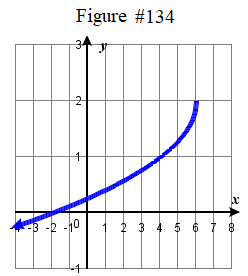135.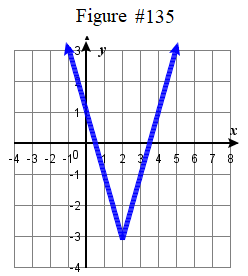136.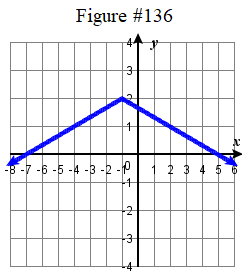137.138.139.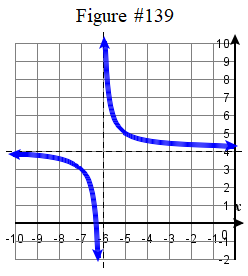131. $$f ( x ) = \tfrac{1}{2} \sqrt { x +3 }$$ 133. $$f ( x ) = \sqrt(2x-5)$$ 135. $$f ( x ) = 2 | x-2 | - 3$$ 137. $$f ( x ) = -\tfrac{1}{2} ( x + 2 )^3 +4$$ 139. $$f(x) = \dfrac{1}{x+6} + 4$$

### G: Construct a formula from a description

Exercise $$\PageIndex{G}$$

$$\bigstar$$ Write a formula for the function with the following transformations

141. Write a formula for the function obtained when the graph of $$f(x)=|x|$$ is shifted down $$3$$ units and right $$1$$ unit.

142. Write a formula for the function obtained when the graph of $$f(x)=\dfrac{1}{x}$$ is shifted down $$4$$ units and right $$3$$ units.

143. Write a formula for the function obtained when the graph of $$f(x)=\dfrac{1}{x^2}$$ is shifted up $$2$$ units and left $$4$$ units.

144. Write a formula for the function obtained when the graph of $$f(x)=\sqrt{x}$$ is shifted up $$1$$ unit and left $$2$$ units.

145. The graph of $$f(x)=|x|$$ is reflected over the $$y$$-axis and horizontally compressed by a factor of $$\dfrac{1}{4}$$.

146. The graph of $$f(x)=\sqrt{x}$$ is reflected over the $$x$$-axis and horizontally stretched by a factor of $$2$$.

147. The graph of $$f(x)=\dfrac{1}{x^2}$$ is vertically compressed by a factor of $$\dfrac{1}{3}$$, then shifted left $$2$$ units and down $$3$$ units.

148. The graph of $$f(x)=\dfrac{1}{x}$$ is vertically stretched by a factor of $$8$$, then shifted to the right $$4$$ units and up $$2$$ units.

149. The graph of $$f(x)=x^2$$ is vertically compressed by a factor of $$\dfrac{1}{2}$$, then shifted to the right $$5$$ units and up $$1$$ unit.

150. The graph of $$f(x)=x^2$$ is horizontally stretched by a factor of $$3$$, then shifted left $$4$$ units and down $$3$$ units.

 141. $$g(x)=|x-1|−3$$ 143. $$g(x)=\dfrac{1}{(x+4)^2}+2$$ 145. $$g(x)=|−4x|$$ 147. $$g(x)=\dfrac{1}{3(x+2)^2}−3$$ 149. $$g(x)=\dfrac{1}{2}(x-5)^2+1$$

### H: Construct equations from transformations of tabular values

Exercise $$\PageIndex{H}$$

$$\bigstar$$ Given tabular representations for the functions $$f$$, $$g$$, and $$h$$, write $$g(x)$$ and $$h(x)$$ as transformations of $$f(x)$$.

155. Tabular representations for the functions $$f$$, $$g$$, and $$h$$ are given below. Write $$g(x)$$ and $$h(x)$$ as transformations of $$f(x)$$.

 $$f(x)$$: $$x$$: -2 -1 0 1 2 -2 -1 -3 1 2
 $$x$$: $$g(x)$$: -1 0 1 2 3 -2 -1 -3 1 2
 $$x$$: $$h(x)$$: -2 -1 0 1 2 -1 0 -2 2 3

156. Tabular representations for the functions $$f$$, $$g$$, and $$h$$ are given below. Write $$g(x)$$ and $$h(x)$$ as transformations of $$f(x)$$.

 $$f(x)$$: $$x$$: -2 -1 0 1 2 -1 -3 4 2 1
 $$x$$: $$g(x)$$: -3 -2 -1 0 1 -1 -3 4 2 1
 $$h(x)$$: $$x$$: -2 -1 0 1 2 -2 -4 3 1 0

155. $$g(x)=f(x-1)$$, $$h(x)=f(x)+1$$.

### I: Identify Increasing/decreasing Intervals

Exercise $$\PageIndex{I}$$

$$\bigstar$$ Use transformations to determine the interval(s) on which the function is increasing and decreasing.

 151. $$g(x)=5(x+3)^2−2$$ 152. $$f(x)=4(x+1)^2−5$$ 153. $$k(x)=−3\sqrt{x}−1$$ 154. $$a(x)=\sqrt{−x+4}$$
 151. decreasing on $$(−\infty,−3)$$ and increasing on $$(−3,\infty)$$ 153. decreasing on $$(0, \infty)$$
$$\star$$